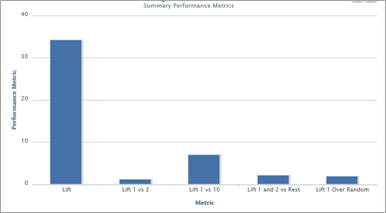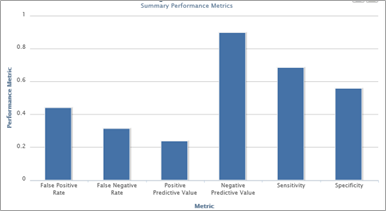# Performance Analysis: Metrics to Analyze: Classification Models

Classification Models:   The following models all make available the same performance output.

·         Binary Classification

·         Affinity

·         Product Affinity

·         Churn

·         Response

·         RiskLift - An overall measure of the model’s sorting efficiency of targets and can range in value from 0 to 100 with 0 being no better than random.  If the model were perfect all of the targets would get assigned higher scores than all of the non-targets and the lift would be 100.

Lift 1 vs. 2 – The performance of decile 1 as compared to decile 2 expressed in the form of an index.  For example a value of 1.2586 indicates decile 1 performance is nearly 26% better than decile 2.

Lift 1 vs. 10 - The performance of decile 1 as compared to decile 10 expressed in the form of an index.  For example a value of 7.1257 indicates decile 1 performance 7x better than decile 10.

Lift 1 and 2 vs. Rest – The performance of deciles 1 and 2 combined as compared to deciles 3-10 combined.  For example a value of 2.2249 indicates deciles 1 and 2 combined performance 2.2x better than the bottom 8 deciles.

Lift 1 Over Random – The performance of decile 1 as compared to the population total.  For example a value of 1.9918 indicates decile 1 performance is 99% better or nearly 2x the population total and if you were to select randomly.

Ranked Value Correlation* = uses the ranking of the model scores from best to worst and calculates the correlation coefficient to the actual occurrences.  The value will always be between 0 and 1 with higher values better.  * This was not selected for the graph because the scale of the value is much lower than everything else and so the bar for this would not be visible.The key to understanding these is understanding the numerator and denominators for each metric.  The idea behind these come from the matrix below.  A prediction is categorized as a 1 if the prediction is equal to or greater than the population rate.  For example if the population response rate is .015 (1.5%) and the model prediction/score is .016 then that record has a predicted result of 1 whereas a record with a prediction/score of say .01 would have a predicted result of 0.

 Actual Result 1 0 Predicted Result 1 True Positives (a) False Positives (b) 0 False Negatives (c) True Negatives (d)

False Positive Rate = b / (b+d) what percent of the actual negatives were incorrectly predicted

False Negative Rate = c / (a+b) what percent of the actual positives were incorrectly predicted

Positive Predictive Value = a / (a+b) what percent of the predicted positives were correct

Negative Predictive Value = c / (c+d) what percent of the predicted negatives were correct

Sensitivity (True Positive Rate) = a / (a+c) what percent of the actual positives were correctly predicted

Specificity (True Negative Rate) = d / (b+d) what percent of the actual negatives were correctly predicted

Percent Correct* = (a+d) / (a+b+c+d) = what percent of the models predictions were correct.  * This was not selected for the graph because the scale of the value is much higher than anything else and so the other bars appear too small to read.

#### Other Articles